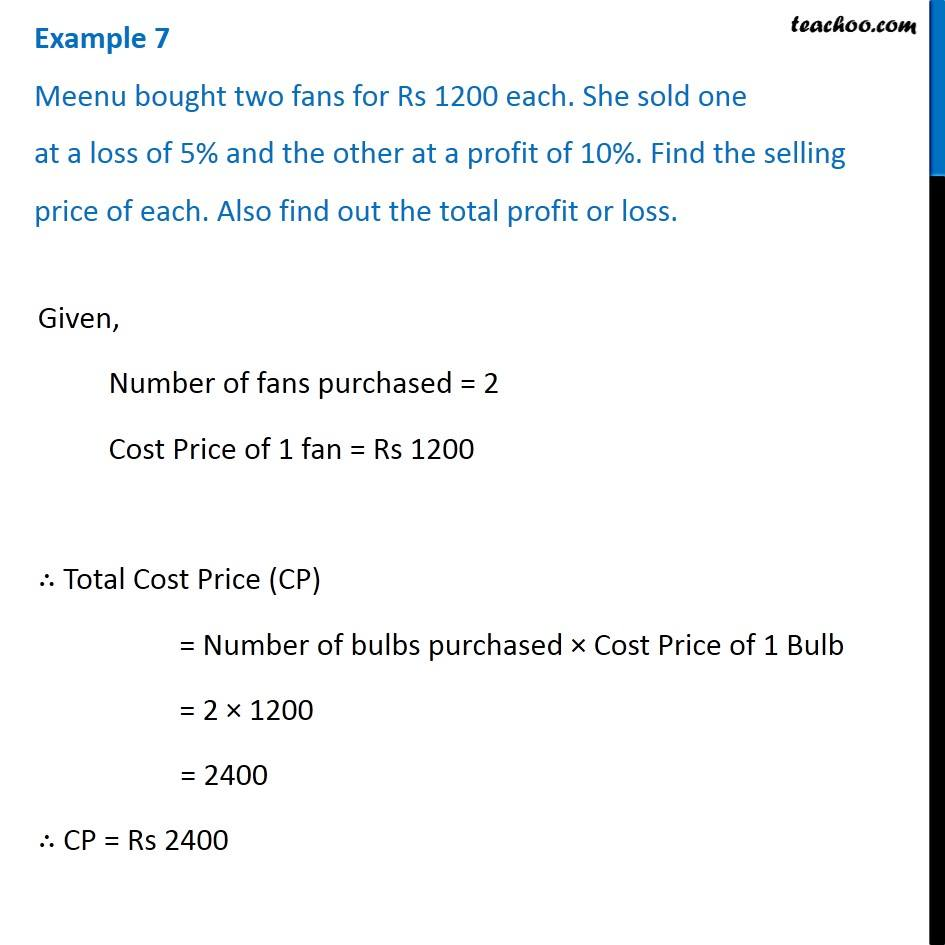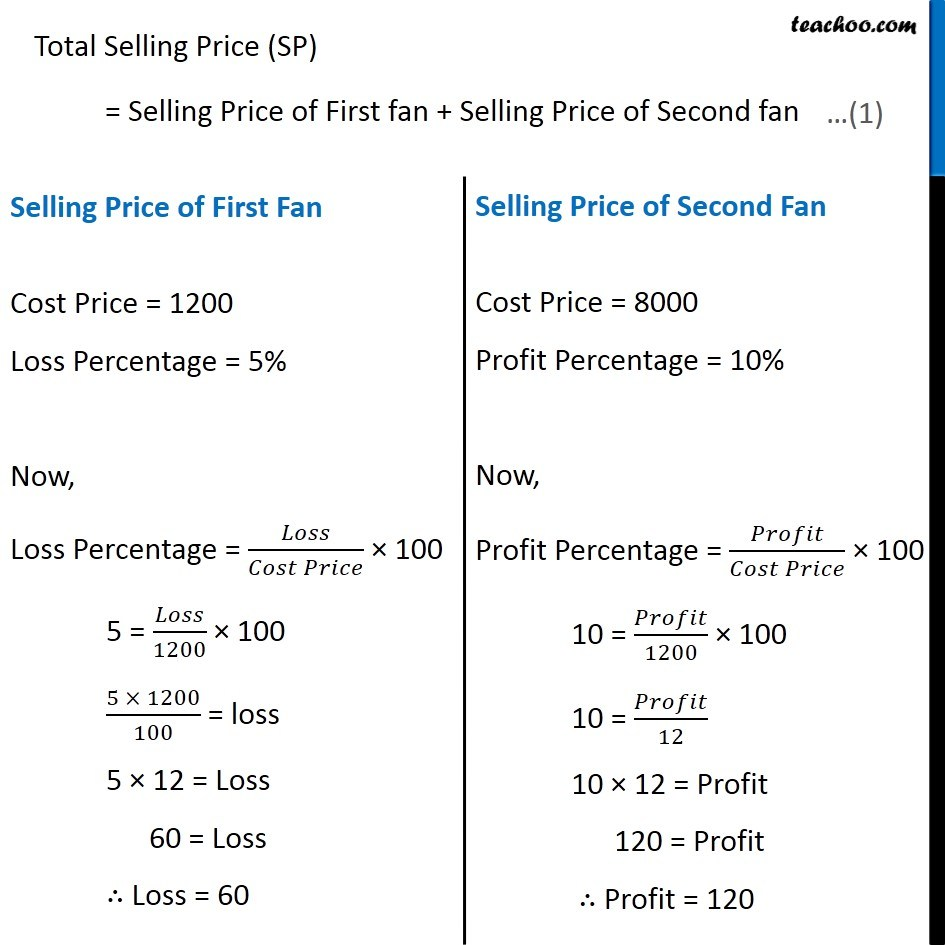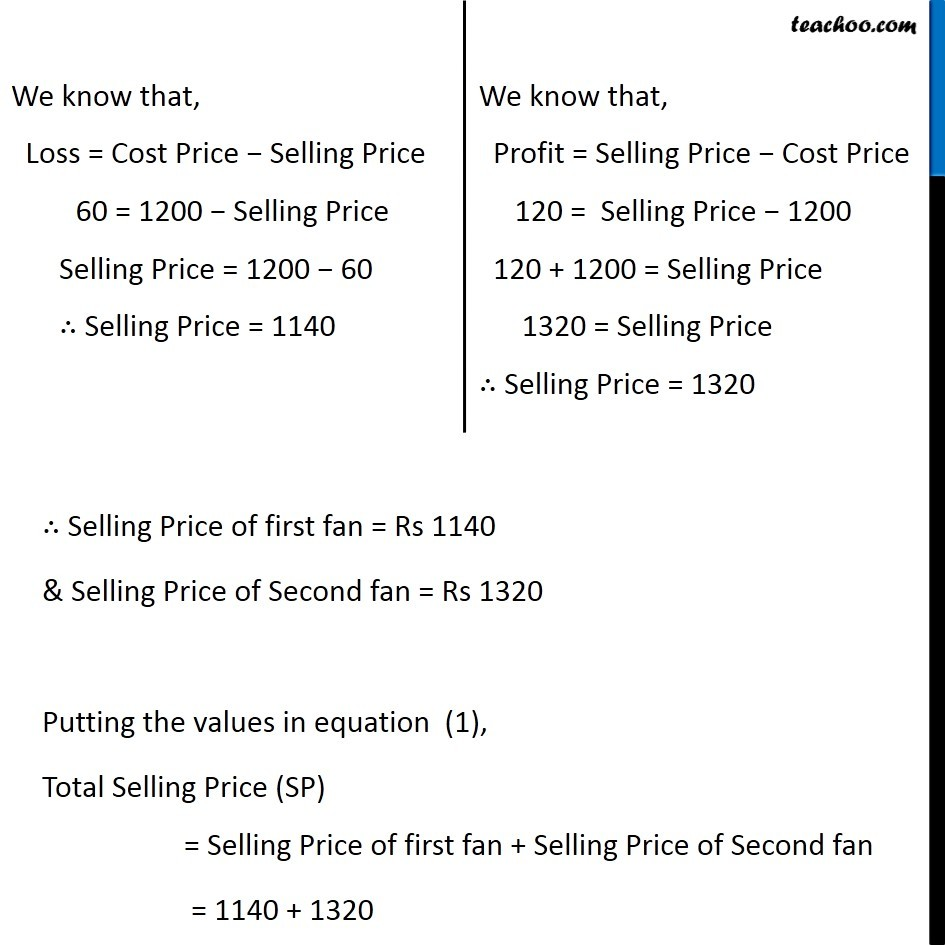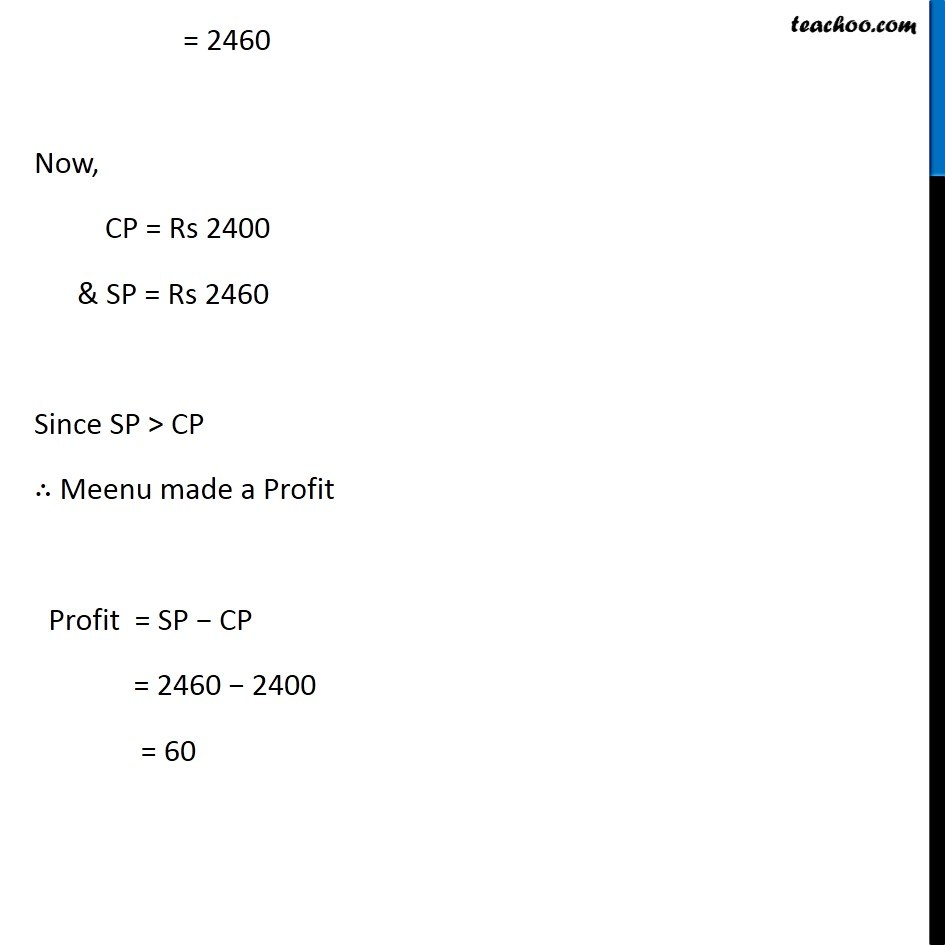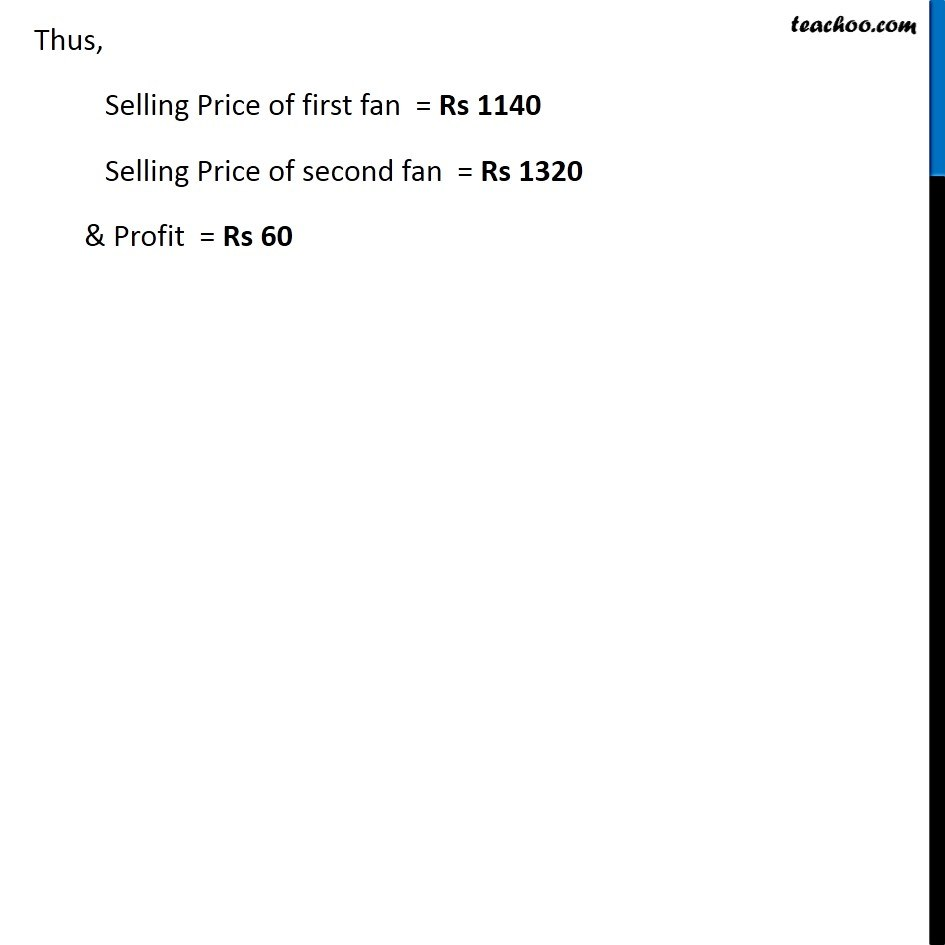1. Chapter 8 Class 8 Comparing Quantities
2. Concept wise
3. Finding cost price, selling price, profit % , loss%

Transcript

Example 7 Meenu bought two fans for Rs 1200 each. She sold one at a loss of 5% and the other at a profit of 10%. Find the selling price of each. Also find out the total profit or loss. Given, Number of fans purchased = 2 Cost Price of 1 fan = Rs 1200 ∴ Total Cost Price (CP) = Number of bulbs purchased × Cost Price of 1 Bulb = 2 × 1200 = 2400 ∴ CP = Rs 2400 Total Selling Price (SP) = Selling Price of First fan + Selling Price of Second fan Selling Price of First Fan Cost Price = 1200 Loss Percentage = 5% Now, Loss Percentage = 𝐿𝑜𝑠𝑠/(𝐶𝑜𝑠𝑡 𝑃𝑟𝑖𝑐𝑒) × 100 5 = 𝐿𝑜𝑠𝑠/1200 × 100 (5 × 1200)/100 = loss 5 × 12 = Loss 60 = Loss ∴ Loss = 60 Selling Price of Second Fan Cost Price = 8000 Profit Percentage = 10% Now, Profit Percentage = 𝑃𝑟𝑜𝑓𝑖𝑡/(𝐶𝑜𝑠𝑡 𝑃𝑟𝑖𝑐𝑒) × 100 10 = 𝑃𝑟𝑜𝑓𝑖𝑡/1200 × 100 10 = 𝑃𝑟𝑜𝑓𝑖𝑡/12 10 × 12 = Profit 120 = Profit ∴ Profit = 120 We know that, Loss = Cost Price − Selling Price 60 = 1200 − Selling Price Selling Price = 1200 − 60 ∴ Selling Price = 1140 We know that, Profit = Selling Price − Cost Price 120 = Selling Price − 1200 120 + 1200 = Selling Price 1320 = Selling Price ∴ Selling Price = 1320 ∴ Selling Price of first fan = Rs 1140 & Selling Price of Second fan = Rs 1320 Putting the values in equation (1), Total Selling Price (SP) = Selling Price of first fan + Selling Price of Second fan = 1140 + 1320 = 2460 Now, CP = Rs 2400 & SP = Rs 2460 Since SP > CP ∴ Meenu made a Profit Profit = SP − CP = 2460 − 2400 = 60 Thus, Selling Price of first fan = Rs 1140 Selling Price of second fan = Rs 1320 & Profit = Rs 60

Finding cost price, selling price, profit % , loss%

Chapter 8 Class 8 Comparing Quantities
Concept wise